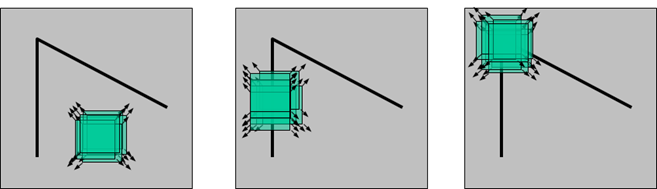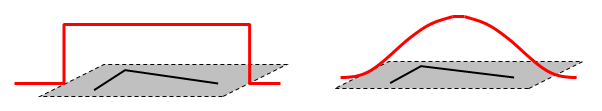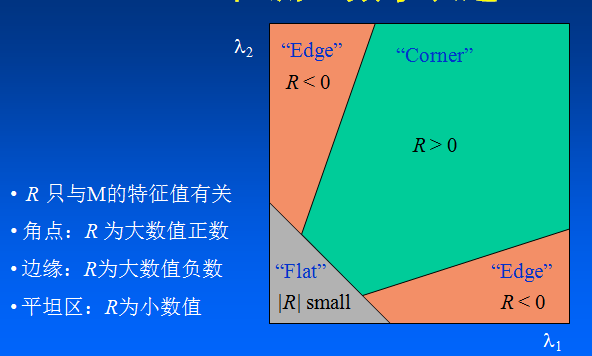Harris角点检测onlyloveyd 74 4

## 什么是角点？

• 灰度梯度的最大值对应的像素点；
• 两条直线或者曲线的交点；
• 一阶梯度的导数最大值和梯度方向变化率最大的像素点；
• 一阶导数最大，二阶导数为零的像素点（指示物体边缘变化不连续的方向）。

## Harris角点检测Harris角点检测原理用公式演算如下： $$E(u,v)= \sum_{x,y}w(x,y)[I(x+u,y+v)-I(x,y)]^2 \ =\sum_{x,y}w(x,y)[I(x,y)+I_xu+I_yv+O(u^2,v^2)-I(x,y)]^2 \ \approx\sum_{x,y}w(x,y)[I_xu+I_yv]^2 \ =\sum_{x,y}w(x,y)[u^2I_x^2+2uvI_xI_y+v^2I_y^2]\ =\sum_{x,y}w(x,y)\begin{bmatrix}u & v\end{bmatrix}\begin{bmatrix} I_x^2 & I_xIy \ I_xI_y & I_y^2\end{bmatrix}\begin{bmatrix}u \ v\end{bmatrix}\ =\begin{bmatrix}u & v\end{bmatrix}(\sum_{x,y}w(x,y)\begin{bmatrix} I_x^2 & I_xIy \ I_xI_y & I_y^2\end{bmatrix})\begin{bmatrix}u \ v\end{bmatrix}$$ 用M代替中间内容： $$E(u,v)=\begin{bmatrix}u & v\end{bmatrix}M\begin{bmatrix}u \ v\end{bmatrix}$$ 其中 $$M=\sum_{x,y}w(x,y)\begin{bmatrix} I_x^2 & I_xIy \ I_xI_y & I_y^2\end{bmatrix}\ I_x,I_y分别为窗口内像素点(x,y)在x方向上和y方向上的梯度值。$$ 其中w(x,y)表示滑动窗口权重函数，可以是常数也可以是高斯函数。E(u,v)表示滑动窗口向各个方向移动时像素值衡量系数的变化。$$M=\sum\limits_{(x,y)€W}w(x,y)\begin{bmatrix} I_x^2 & I_xIy \ I_xI_y & I_y^2\end{bmatrix}=\begin{bmatrix} A & C \ C & B \end{bmatrix}$$ E(u,v)可以改写成： $$E(u,v)=Au^2+2Cuv+Bv^2\ A=\sum\limits_{(x,y)€W}w(x,y)I_x^2 \ B=\sum\limits_{(x,y)€W}w(x,y)I_y^2 \ C=\sum\limits_{(x,y)€W}w(x,y)*I_xI_y \$$## API

public static void cornerHarris(Mat src, Mat dst, int blockSize, int ksize, double k, int borderType) 
• 参数一：src，输入源图像。必须是单通道8U或者32F类型。
• 参数二：dst，输出评价系数R的矩阵。尺寸与src相同，类型为单通道32F。
• 参数三：blockSize，邻域大小。
• 参数四：ksize，Sobel算子的半径。
• 参数五：k，计算Harris评价系数R的权重系统。
• 参数六：borderType，像素外推算法标志位。

## 操作

/**
* Harris角点检测
* author: yidong
* 2020/12/30
*/
class HarrisActivity : AppCompatActivity() {
private val mBinding: ActivityHarrisBinding by lazy {
ActivityHarrisBinding.inflate(layoutInflater)
}

private val gray by lazy {
this.getBgrFromResId(R.drawable.lena).toGray()
}

override fun onCreate(savedInstanceState: Bundle?) {
super.onCreate(savedInstanceState)
setContentView(mBinding.root)

mBinding.ivLena.showMat(gray)
wrapCoroutine({ showLoading() }, { doCornerHarris() }, { hideLoading() })
}

private fun doCornerHarris() {
val dst = Mat()
val dstNorm = Mat()
val dstNormal8U = Mat()
Imgproc.cornerHarris(gray, dst, 2, 3, 0.04)
Core.normalize(dst, dstNorm, 0.0, 255.0, Core.NORM_MINMAX)
Core.convertScaleAbs(dstNorm, dstNormal8U)
Imgproc.threshold(dstNormal8U, dstNormal8U, 120.0, 255.0, Imgproc.THRESH_BINARY)
GlobalScope.launch(Dispatchers.Main) {
mBinding.ivResult.showMat(dstNormal8U)
}
}

private fun showLoading() {
mBinding.isLoading = true
}

private fun hideLoading() {
mBinding.isLoading = false
}

override fun onDestroy() {
super.onDestroy()
gray.release()
}
}

## 效果## 源码

https://www.helloworld.net/redirect?target=https://github.com/onlyloveyd/LearningAndroidOpenCV